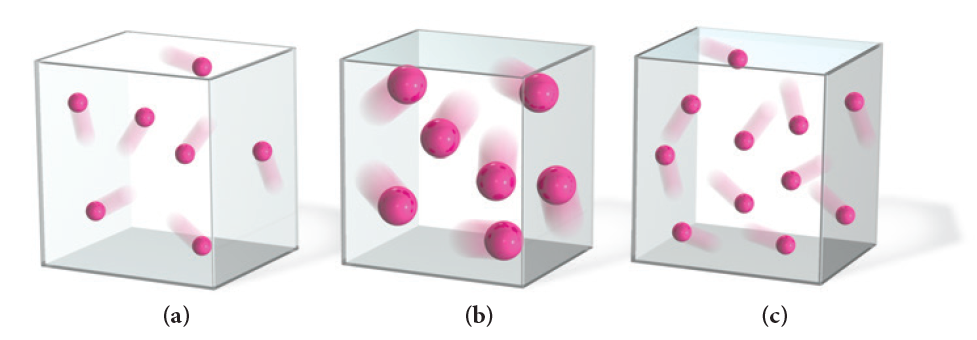# Problem: Which sample of an ideal gas has the greatest pressure? Assume that the mass of each particle is proportional to its size and that all the gas samples are at the same temperature.

###### FREE Expert Solution

Recall that the ideal gas law is:

$\overline{){\mathbf{PV}}{\mathbf{=}}{\mathbf{nRT}}}$

93% (172 ratings)###### Problem Details

Which sample of an ideal gas has the greatest pressure? Assume that the mass of each particle is proportional to its size and that all the gas samples are at the same temperature.